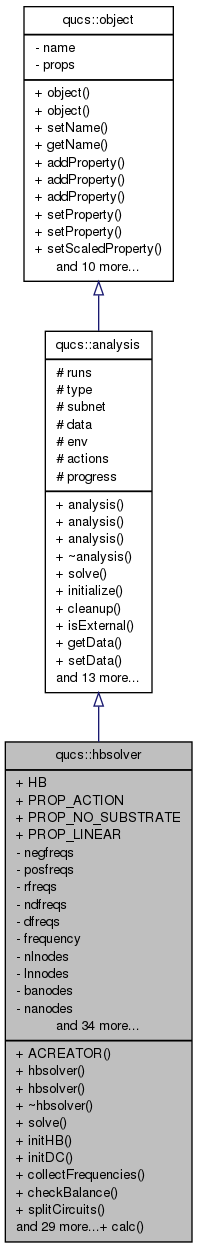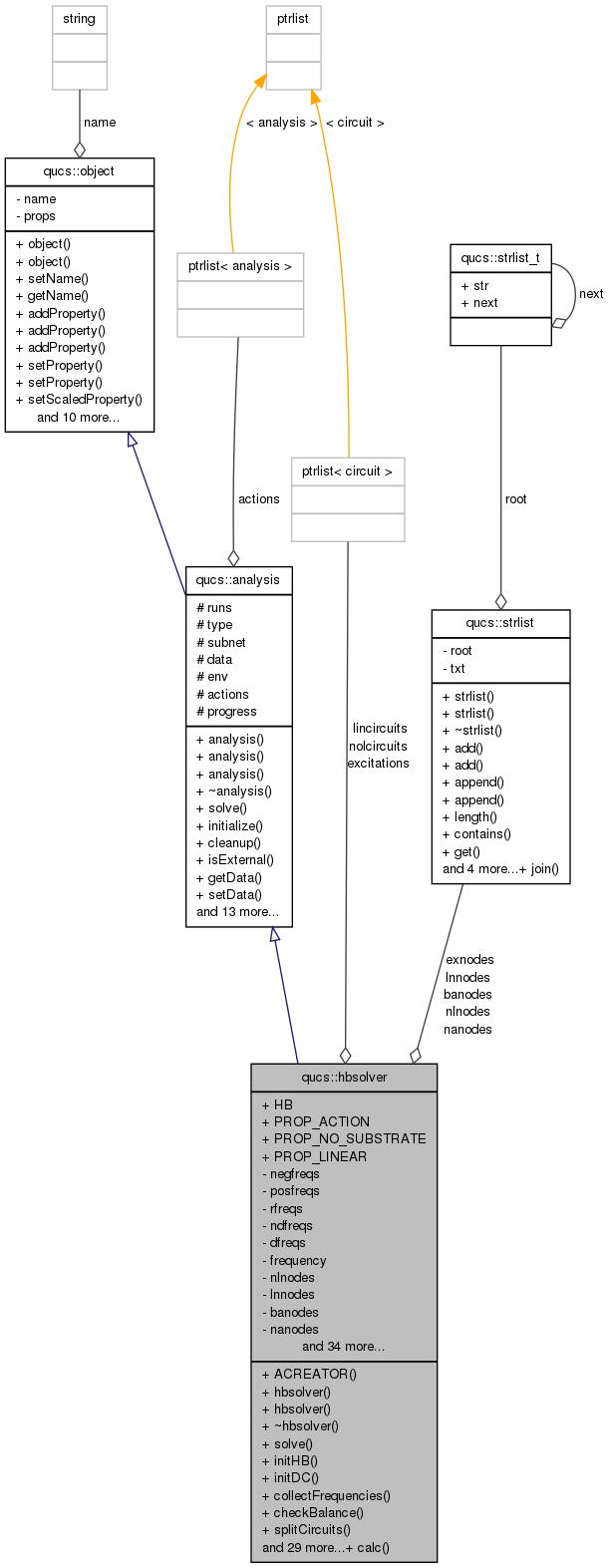Qucs-core  0.0.19
qucs::hbsolver Class Reference

`#include <hbsolver.h>`

Inheritance diagram for qucs::hbsolver:[legend]
Collaboration diagram for qucs::hbsolver:[legend]

## Public Member Functions

ACREATOR (hbsolver)
hbsolver (char *)
hbsolver (hbsolver &)
~hbsolver ()
int solve (void)
placehoder for solution function
void initHB (void)
void initDC (void)
void collectFrequencies (void)
int checkBalance (void)
void splitCircuits (void)
void expandFrequencies (nr_double_t, int)
bool isExcitation (circuit *)
strlistcircuitNodes (ptrlist< circuit >)
void getNodeLists (void)
int assignVoltageSources (ptrlist< circuit >)
int assignNodes (ptrlist< circuit >, strlist *, int offset=0)
void prepareLinear (void)
void createMatrixLinearA (void)
void fillMatrixLinearA (tmatrix< nr_complex_t > *, int)
void invertMatrix (tmatrix< nr_complex_t > *, tmatrix< nr_complex_t > *)
void createMatrixLinearY (void)
void saveResults (void)
void calcConstantCurrent (void)
nr_complex_t excitationZ (tvector< nr_complex_t > *, circuit *, int)
void finalSolution (void)
void fillMatrixNonLinear (tmatrix< nr_complex_t > *, tmatrix< nr_complex_t > *, tvector< nr_complex_t > *, tvector< nr_complex_t > *, tvector< nr_complex_t > *, tvector< nr_complex_t > *, int)
void prepareNonLinear (void)
void solveHB (void)
void loadMatrices (void)
void VectorFFT (tvector< nr_complex_t > *, int isign=1)
void VectorIFFT (tvector< nr_complex_t > *, int isign=1)
int calcOrder (int)
void MatrixFFT (tmatrix< nr_complex_t > *)
void calcJacobian (void)
void solveVoltages (void)
tvector< nr_complex_texpandVector (tvector< nr_complex_t >, int)
tmatrix< nr_complex_texpandMatrix (tmatrix< nr_complex_t >, int)
tmatrix< nr_complex_textendMatrixLinear (tmatrix< nr_complex_t >, int)
void fillMatrixLinearExtended (tmatrix< nr_complex_t > *, tvector< nr_complex_t > *)
void saveNodeVoltages (circuit *, int)

## Static Public Member Functions

static void calc (hbsolver *)

## Data Fields

HB
PROP_ACTION
PROP_NO_SUBSTRATE
PROP_LINEAR

## Private Attributes

std::vector< nr_double_tnegfreqs
std::vector< nr_double_tposfreqs
std::vector< nr_double_trfreqs
intndfreqs
std::vector< nr_double_tdfreqs
nr_double_t frequency
strlistnlnodes
strlistlnnodes
strlistbanodes
strlistnanodes
strlistexnodes
ptrlist< circuitexcitations
ptrlist< circuitnolcircuits
ptrlist< circuitlincircuits
tmatrix< nr_complex_t > * Y
tmatrix< nr_complex_t > * A
tmatrix< nr_complex_t > * Z
tmatrix< nr_complex_t > * YV
tmatrix< nr_complex_t > * NA
tmatrix< nr_complex_t > * JQ
tmatrix< nr_complex_t > * JG
tmatrix< nr_complex_t > * JF
tvector< nr_complex_t > * IG
tvector< nr_complex_t > * FQ
tvector< nr_complex_t > * VS
tvector< nr_complex_t > * VP
tvector< nr_complex_t > * FV
tvector< nr_complex_t > * IL
tvector< nr_complex_t > * IN
tvector< nr_complex_t > * IR
tvector< nr_complex_t > * QR
tvector< nr_complex_t > * RH
tvector< nr_complex_t > * OM
tvector< nr_complex_t > * IC
tvector< nr_complex_t > * IS
tvector< nr_complex_t > * x
tvector< nr_complex_t > * vs
int runs
int lnfreqs
int nlfreqs
int nnlvsrcs
int nlnvsrcs
int nnanodes
int nexnodes
int nbanodes

## Detailed Description

Definition at line 1429 of file hbsolver.cpp.

## Constructor & Destructor Documentation

 qucs::hbsolver::hbsolver ( char * n )

Definition at line 72 of file hbsolver.cpp.

 qucs::hbsolver::hbsolver ( hbsolver & o )

Definition at line 126 of file hbsolver.cpp.

 qucs::hbsolver::~hbsolver ( )

Definition at line 85 of file hbsolver.cpp.

## Member Function Documentation

 qucs::hbsolver::ACREATOR ( hbsolver )
 int qucs::hbsolver::assignNodes ( ptrlist< circuit > circuits, strlist * nodes, int offset = `0` )

Definition at line 532 of file hbsolver.cpp.

 int qucs::hbsolver::assignVoltageSources ( ptrlist< circuit > circuits )

Definition at line 520 of file hbsolver.cpp.

 void qucs::hbsolver::calc ( hbsolver * self ) ` [static]`

Definition at line 300 of file hbsolver.cpp.

 void qucs::hbsolver::calcConstantCurrent ( void )

Definition at line 874 of file hbsolver.cpp.

 void qucs::hbsolver::calcJacobian ( void )

Definition at line 1208 of file hbsolver.cpp.

 int qucs::hbsolver::calcOrder ( int n )

Definition at line 367 of file hbsolver.cpp.

 int qucs::hbsolver::checkBalance ( void )

Definition at line 928 of file hbsolver.cpp.

 strlist * qucs::hbsolver::circuitNodes ( ptrlist< circuit > circuits )

Definition at line 464 of file hbsolver.cpp.

 void qucs::hbsolver::collectFrequencies ( void )

Definition at line 376 of file hbsolver.cpp.

 void qucs::hbsolver::createMatrixLinearA ( void )

Definition at line 569 of file hbsolver.cpp.

 void qucs::hbsolver::createMatrixLinearY ( void )

Definition at line 716 of file hbsolver.cpp.

 nr_complex_t qucs::hbsolver::excitationZ ( tvector< nr_complex_t > * V, circuit * vs, int f )

Definition at line 860 of file hbsolver.cpp.

 void qucs::hbsolver::expandFrequencies ( nr_double_t f, int n )

Definition at line 334 of file hbsolver.cpp.

 tmatrix< nr_complex_t > qucs::hbsolver::expandMatrix ( tmatrix< nr_complex_t > M, int nodes )

Definition at line 1249 of file hbsolver.cpp.

 tvector< nr_complex_t > qucs::hbsolver::expandVector ( tvector< nr_complex_t > V, int nodes )

Definition at line 1228 of file hbsolver.cpp.

 tmatrix< nr_complex_t > qucs::hbsolver::extendMatrixLinear ( tmatrix< nr_complex_t > M, int nodes )

Definition at line 1302 of file hbsolver.cpp.

 void qucs::hbsolver::fillMatrixLinearA ( tmatrix< nr_complex_t > * A, int f )

Definition at line 603 of file hbsolver.cpp.

 void qucs::hbsolver::fillMatrixLinearExtended ( tmatrix< nr_complex_t > * Y, tvector< nr_complex_t > * I )

Definition at line 1318 of file hbsolver.cpp.

 void qucs::hbsolver::fillMatrixNonLinear ( tmatrix< nr_complex_t > * jg, tmatrix< nr_complex_t > * jq, tvector< nr_complex_t > * ig, tvector< nr_complex_t > * fq, tvector< nr_complex_t > * ir, tvector< nr_complex_t > * qr, int f )

Definition at line 964 of file hbsolver.cpp.

 void qucs::hbsolver::finalSolution ( void )

Definition at line 1349 of file hbsolver.cpp.

 void qucs::hbsolver::getNodeLists ( void )

Definition at line 478 of file hbsolver.cpp.

 void qucs::hbsolver::initDC ( void )

Definition at line 318 of file hbsolver.cpp.

 void qucs::hbsolver::initHB ( void )

Definition at line 309 of file hbsolver.cpp.

 void qucs::hbsolver::invertMatrix ( tmatrix< nr_complex_t > * A, tmatrix< nr_complex_t > * H )

Definition at line 654 of file hbsolver.cpp.

 bool qucs::hbsolver::isExcitation ( circuit * c )

Definition at line 326 of file hbsolver.cpp.

 void qucs::hbsolver::loadMatrices ( void )

Definition at line 1072 of file hbsolver.cpp.

 void qucs::hbsolver::MatrixFFT ( tmatrix< nr_complex_t > * M )

Definition at line 1130 of file hbsolver.cpp.

 void qucs::hbsolver::prepareLinear ( void )

Definition at line 551 of file hbsolver.cpp.

 void qucs::hbsolver::prepareNonLinear ( void )

Definition at line 995 of file hbsolver.cpp.

 void qucs::hbsolver::saveNodeVoltages ( circuit * cir, int f )

Definition at line 1060 of file hbsolver.cpp.

 void qucs::hbsolver::saveResults ( void )

Definition at line 1395 of file hbsolver.cpp.

 int qucs::hbsolver::solve ( void ) ` [virtual]`

placehoder for solution function

Virtual solution function intended to be overridden by the inheiriting class's solution function.

Reimplemented from qucs::analysis.

Definition at line 147 of file hbsolver.cpp.

 void qucs::hbsolver::solveHB ( void )

Definition at line 1177 of file hbsolver.cpp.

 void qucs::hbsolver::solveVoltages ( void )

Definition at line 1275 of file hbsolver.cpp.

 void qucs::hbsolver::splitCircuits ( void )

Definition at line 445 of file hbsolver.cpp.

 void qucs::hbsolver::VectorFFT ( tvector< nr_complex_t > * V, int isign = `1` )

Definition at line 1096 of file hbsolver.cpp.

 void qucs::hbsolver::VectorIFFT ( tvector< nr_complex_t > * V, int isign = `1` )

Definition at line 1124 of file hbsolver.cpp.

## Field Documentation

 tmatrix* qucs::hbsolver::A` [private]`

Definition at line 102 of file hbsolver.h.

 strlist * qucs::hbsolver::banodes` [private]`

Definition at line 96 of file hbsolver.h.

 std::vector qucs::hbsolver::dfreqs` [private]`

Definition at line 94 of file hbsolver.h.

 ptrlist qucs::hbsolver::excitations` [private]`

Definition at line 97 of file hbsolver.h.

 strlist * qucs::hbsolver::exnodes` [private]`

Definition at line 96 of file hbsolver.h.

 tvector* qucs::hbsolver::FQ` [private]`

Definition at line 112 of file hbsolver.h.

 nr_double_t qucs::hbsolver::frequency` [private]`

Definition at line 95 of file hbsolver.h.

 tvector* qucs::hbsolver::FV` [private]`

Definition at line 115 of file hbsolver.h.

 qucs::hbsolver::HB

Definition at line 1430 of file hbsolver.cpp.

 tvector* qucs::hbsolver::IC` [private]`

Definition at line 124 of file hbsolver.h.

 tvector* qucs::hbsolver::IG` [private]`

Definition at line 111 of file hbsolver.h.

 tvector* qucs::hbsolver::IL` [private]`

Definition at line 116 of file hbsolver.h.

 tvector* qucs::hbsolver::IN` [private]`

Definition at line 117 of file hbsolver.h.

 tvector* qucs::hbsolver::IR` [private]`

Definition at line 119 of file hbsolver.h.

 tvector* qucs::hbsolver::IS` [private]`

Definition at line 125 of file hbsolver.h.

 tmatrix* qucs::hbsolver::JF` [private]`

Definition at line 110 of file hbsolver.h.

 tmatrix* qucs::hbsolver::JG` [private]`

Definition at line 109 of file hbsolver.h.

 tmatrix* qucs::hbsolver::JQ` [private]`

Definition at line 108 of file hbsolver.h.

 ptrlist qucs::hbsolver::lincircuits` [private]`

Definition at line 99 of file hbsolver.h.

 int qucs::hbsolver::lnfreqs` [private]`

Definition at line 130 of file hbsolver.h.

 strlist * qucs::hbsolver::lnnodes` [private]`

Definition at line 96 of file hbsolver.h.

 tmatrix* qucs::hbsolver::NA` [private]`

Definition at line 106 of file hbsolver.h.

 strlist * qucs::hbsolver::nanodes` [private]`

Definition at line 96 of file hbsolver.h.

 int qucs::hbsolver::nbanodes` [private]`

Definition at line 137 of file hbsolver.h.

 int* qucs::hbsolver::ndfreqs` [private]`

Definition at line 93 of file hbsolver.h.

 std::vector qucs::hbsolver::negfreqs` [private]`

Definition at line 90 of file hbsolver.h.

 int qucs::hbsolver::nexnodes` [private]`

Definition at line 136 of file hbsolver.h.

 int qucs::hbsolver::nlfreqs` [private]`

Definition at line 131 of file hbsolver.h.

 strlist* qucs::hbsolver::nlnodes` [private]`

Definition at line 96 of file hbsolver.h.

 int qucs::hbsolver::nlnvsrcs` [private]`

Definition at line 133 of file hbsolver.h.

 int qucs::hbsolver::nnanodes` [private]`

Definition at line 135 of file hbsolver.h.

 int qucs::hbsolver::nnlvsrcs` [private]`

Definition at line 132 of file hbsolver.h.

 ptrlist qucs::hbsolver::nolcircuits` [private]`

Definition at line 98 of file hbsolver.h.

 tvector* qucs::hbsolver::OM` [private]`

Definition at line 122 of file hbsolver.h.

 std::vector qucs::hbsolver::posfreqs` [private]`

Definition at line 91 of file hbsolver.h.

Definition at line 1430 of file hbsolver.cpp.

Definition at line 1430 of file hbsolver.cpp.

Definition at line 1430 of file hbsolver.cpp.

 tvector* qucs::hbsolver::QR` [private]`

Definition at line 120 of file hbsolver.h.

 std::vector qucs::hbsolver::rfreqs` [private]`

Definition at line 92 of file hbsolver.h.

 tvector* qucs::hbsolver::RH` [private]`

Definition at line 121 of file hbsolver.h.

 int qucs::hbsolver::runs` [private]`

Reimplemented from qucs::analysis.

Definition at line 129 of file hbsolver.h.

 tvector* qucs::hbsolver::VP` [private]`

Definition at line 114 of file hbsolver.h.

 tvector* qucs::hbsolver::VS` [private]`

Definition at line 113 of file hbsolver.h.

 tvector* qucs::hbsolver::vs` [private]`

Definition at line 127 of file hbsolver.h.

 tvector* qucs::hbsolver::x` [private]`

Definition at line 126 of file hbsolver.h.

 tmatrix* qucs::hbsolver::Y` [private]`

Definition at line 101 of file hbsolver.h.

 tmatrix* qucs::hbsolver::YV` [private]`

Definition at line 105 of file hbsolver.h.

 tmatrix* qucs::hbsolver::Z` [private]`

Definition at line 103 of file hbsolver.h.

The documentation for this class was generated from the following files: